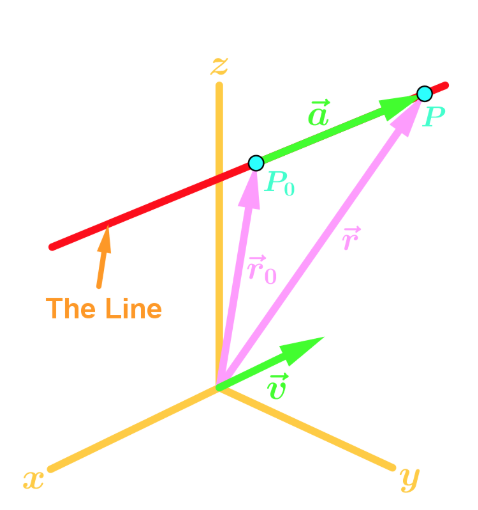# 3-Dimensional lines

### 3-Dimensional lines

#### Lessons

Notes:

Vector Equation for 3-Dimensional Lines
Unlike 2D lines which have the equation $y=mx+b$, 3-Dimensional lines can be expressed as vector functions in the form

$\vec{r(t)} = \vec{r_0} + \vec{tv} = \lt x_0, y_0, z_0 \gt + \; t \lt a,b,c \gt$

where t is a variable. Here is the visual representation of the vector $\vec{r(t)}$.There are 2 other alternate ways to express a 3-Dimensional line.

Parametric Equation
From the equation above, we can rearrange the vector equation so that:

$\vec{r(t)} = \lt x_0,y_0,z_0 \gt + \;t\lt a,b,c \gt$
$\lt x,y,z \gt = \lt x_0, y_0, z_0 \gt + \lt ta,tb,tc \gt$
$= \lt x_0 + ta, y_0 + tb , z_0 + tc \gt$

Then we can see that:

$x = x_0 + ta$
$y = y_0 + tb$
$z = z_0 + tc$

Hence these set of equations of the Parametric Equation of the equation of the 3D line.

Symmetric Equations Assume that $a,b,c$ are non-zero. Then rearranging the set of equations from above gives us:

$x = x_0 + ta \to x - x_0 = ta \to \frac{x-x_0}{a} = t$
$y = y_0 + tb \to y - y_0 = tb \to \to \frac{y-y_0}{b} = t$
$z = z_0 + tc \to z - z_0 = tc \to \frac{z-z_0}{c} = t$

Hence we have our symmetric equation of the equation of the 3D line:

$\frac{x-x_0}{a} = \frac{y-y_0}{b} = \frac{z-z_0}{c}$

• Introduction
3-Dimensional Lines Overview:
a)
Vector Equation 3D lines
• Need a vector equation to express 3D lines
• Diagram for the vector equation
• $\vec{r(t)} = \lt x_0 , y_0, z_0 \gt +\; t \lt a,b,c \gt$

b)
Parametric Equations
• Another way to express 3D lines
• How to get Parametric Equations
• An example

c)
Symmetric Equations
• Another way to express 3D lines
• How to get Symmetric Equations?
• An example

• 1.
Question with Two Points
If the line passes through the points $(2, 1, 0)$ and $(1, 4, 5)$, find the equation of the line in vector form.

• 2.
If the line passes through the points $(-2, 3, 5)$ and $(-1, 0, -3)$, find the equation of the line in symmetric form.

• 3.
Question with a Point and a Line
If the line passes through the point $(0, 1, 3)$ and is parallel to the line $<1-2t, 5+t, -t>$, find the vector equation of the line.

• 4.
Intersection of a Line and a Plane
Determine whether the line given by $<5-t, -5, 1+2t>$ and the $xy$-plane will intersect. If so, find the intersection point.

• 5.
Determine whether the line given by $<5-t, -5, 1+2t>$ and the $xz$-plane will intersect. If so, find the intersection point.